Students get through the MP Board Class 11th Chemistry Important Questions Chapter 9 Hydrogen which are most likely to be asked in the exam.

## MP Board Class 11th Chapter 10 The s-Block Elements

The s-Block Elements Class 11 Important Questions Very Short Answer Type

Question 1.
Why are alkali metals not found in nature ?
Answer:
Alkali metals, due to high chemical reactivity is not found in free state in nature. It is found in earth’s crust in the form of ores like halide, sulphate, carbonate, silicate, borate, oxide etc.

Question 2.
Explain why is sodium less reactive than potassium?
Answer:
Ionisation enthalpy of potassium (ΔiH1) 419 kJ mol-1 is less than ionisation enthalpy (496 kJ mol-1) of sodium and standard electrode potential (- 2.925 V) of potassium is more negative than the corresponding value (- 2.714 V) of sodium. That is why sodium is less reactive than potassium.

Question 3.
Potassium carbonate cannot be prepared by Solvay process. Why?
Answer:
Potassium carbonate cannot be prepared by Solvay process because potassium bicarbonate being soluble in water do not get precipitated.

Question 4.
Why does Li2CO3 decompose at a lower temperature where as Na2CO3 at a higher temperature?
Answer:
Lithium due to its small size extremely polarize CO32- ions and form more stable Li2O and CO2. Carbonates of alkali metals (except Lithium) are stable at high temperature. That is why, Li2CO3 decompose at lower temperature and Na2CO3 at a higher temperature.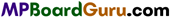Question 5.
Write name and formula of an ore in which both Ca and Mg are present.
Ans. Name of ore: Dolomite
Formula: MgCO3.CaCO3.

Question 6.
Write the name of flux which are used for the removal of acidic impurities in metallurgical processes.
Answer:

1. Limestone CaCO3,
2. Magnesite MgCO3.

Question 7.
Which property of Plaster of Paris is used in plastering?
Answer:
Plaster of Paris reacts with water and becomes hard like cement. Due to this property, it is used in plastering for joining crack bones.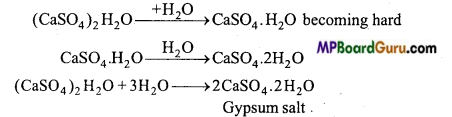Question 8.
IA and IIA groups are known as s-block elements. Why?
Answer:
On writing the electronic configuration of all the elements present in IA and IIA group, the last electron enters the s-subshell, therefore it is known as .y-block element.
Example: Na11 → 1s2,2s2, 2p6,3s1
Ca20 →1s2,2s2,2p6,3s2, 3p6,4s2.

Question 9.
What is the Sorrel cement? For which work is it used?
Answer:
If MgO is mixed in concentrated solution of magnesium chloride then a white paste of magnesium oxy chloride of the composition MgCl2. 2MgO. xH2O is formed which settles and become hard. It is known as Sorrel cement. It is used to fill the cavities in teeth and in joining clay and porcelain utensils.

Question 10.
What changes are obtained on keeping sodium carbonate open in air ? Explain with equation.
Answer:
On keeping Na2CO3.10H2O open in air its water of crystallization slowly evaporates and it gets converted to monohydrate Na2CO3.H2O which is an efflorescent. On heating it to 750°C anhydrous sodium carbonate is formed which is also called soda-ash.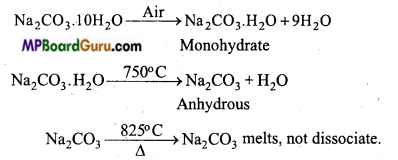Question 11.
Lithium hydride can be used for the preparation of other hydrides. Beryllium hydride is one among them. State the various steps of its preparation. Write the chemical equations used in this process.
Answer:
BeH2 is manufactured by the reduction of complex alkali metal hydrides like Lithium aluminium hydride.
8LiH + Al2Cl6 → 2LiAlH4+6LiCl
2BeCl2 + LiAlH4 →2BeH2 + LiCl + AlCl3.

Question 12.
Determine the oxidation state of sodium in Na2O2.
Answer:
Let oxidation state of Na in Na2O2 bex In Na2O2 (Na-O-O-Na) one peroxide bond is found in which oxidation state of O is -1.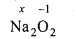or 2x + 2(-1) = 0
or x = + 1
Thus, oxidation state of sodium in Na2O2 is +1.

Question 13.
How is calcium sulphate prepared? Write its use.
Answer:
In the laboratory, on reacting oxide, carbonate, chloride of Ca with dilute H2SO4, calcium sulphate is obtained.
CaO + H2SO4 → CaSO4 + H2O
CaCO3 + H2SO4 → CaSO4 + H2O + CO2

Uses :

1. In preparing chalk,
2. In cement industry,
3. In the preparation of Plaster of Paris,
4. As a fertilizer.

Question 14.
What is Gypsum? How is Plaster of Paris prepared from it?
Answer:
Calcium sulphate (CaSO4.2H2O) is called gypsum.
When heated to 120°C-130°C in absence of hydrocarbon fuel, it loses three fourth of its water content to form Plaster of Paris.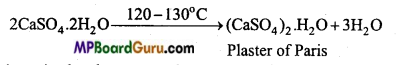Plaster of Paris again absorbs water and get converted to gypsum.

Question 15.
Temperature of furnace is kept less than 1000°C while preparing quick lime. Explain the reason with chemical equation.
Answer:
The temperature of the furnace used to prepare lime is kept less than 1000°C because at high-temperature CaO will react with Silica (SiO2) present as impurity and form fusible silicate.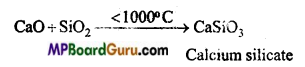Question 16.
Sodium is stored in kerosene oil. Why?
Answer:
Sodium is extremely reactive and strong electropositive element. It reacts with O2 present in the atmosphere, moisture and carbon dioxide to form oxide and hydroxide. Therefore, sodium is stored in keroseiie oil due to which it cannot come in contact with air.
4Na + O2 → 2 Na2O
Na2O + H2O →2NaOH
2NaOH + CO2 →Na2CO3 + H2O.Question 17.
Write the formula of lime water. What changes will take place on passing CO2 in it?
Answer:
Solution of quick lime in water is known as lime water. Its formula is Ca(OH)2.
1. Lime water turns milky on passing CO2 through it due to the formation of CaCO3.
Ca(OH)2 + CO2 → CaCO3 + H2O

2. On passing CO2 in lime water for a long time milkiness of lime water disappears.
CaCO3 +CO2 +H2O → Ca(HCO3)2.

Question 18.
Why can K2CO3 not be prepared by Solvay process?
Answer:
K2CO3 cannot be prepared by Solvay process because KHCO3 analogous to NaHCO3 obtained in between the reaction is soluble due to which it cannot be obtained by crystallization.

Question 19.
Which metal is used in photochemical cell and why?
Answer:
In photochemical cell, potassium and caesium is used because of their low ionization energy.

Question 20.
Why can extraction of sodium by sodium chloride not be done by normal reducing agent?
Answer:
Sodium is a strong reducing agent. It occupies the highest place in electrochemical series. Stronger reducing agents than this is not available, so it cannot be reduced by normal reducing agents. It can be reduced only by electrolysis.

Question 21.
Li and Be have the tendency to form covalent compounds. Explain.
Answer:
Due to small size of Li and Be atoms and their high ionization energy, the electrons of the valence shell are firmly bound to their nuclei. The polarizing power of their ions is also high due to high charge density, hence Li and Be form covalent compounds.

Question 22.
Write down Lewis structure of O2 and write oxidation state of each oxygen atom. What is the average oxidation state of this ion?
Answer:
Lewis structure of O2 ion is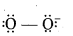Uncharged oxygen atom contains six electrons, thus its oxidation state is zero. But oxygen with -1 charge has 7 electrons, thus its oxidation state is -1.
Oxidation state of each oxygen atom = –$$\frac{1}{2}$$
O2 = 2x = -1
⇒ x = –$$\frac{1}{2}$$

Question 23.
In Solvay process, can we obtain sodium carbonate by directly reacting ammonium carbonate solution with sodium chloride?
Answer:
No, because ammonium carbonate reacts with sodium chloride as follows :
(NH4)2CO3 + 2NaCl⇌Na2CO3 + 2NH4Cl Since the products obtained Na2CO3 and NH4Cl both are more soluble due to which equilibrium does not displace in forward direction. That is why we cannot obtain sodium carbonate by directly reacting ammonium carbonate solution with sodium chloride.Question 24.
All compounds of Alkali metals are soluble in water but why are Lithium compounds more soluble in organic compounds?
Answer:
Due to comparatively small size of Li+ and high polarizing power covalent character is produced in it (Fajan’s Rule). Compounds of other alkali metals are of ionic nature due to which they are soluble in water whereas Lithium compounds due to covalent nature is soluble in alcohol and other organic compounds.

Question 25.
In place of lithium, caesium and potassium is used in photoelectric ceil. Why?
Answer:
Ionization energy of lithium is more than that of potassium and caesium. The elements which possess low ionization energy liberate their electrons easily and are used in photoelectric cells. Thus, potassium and caesium are used in photoelectric cells not lithium.

Question 26.
Beryllium halide produce smoke in air. Why?
Answer:
Normally air contain moisture, thus Beryllium halide hydrolyse in water and release HCl due to which it produces smoke in air.
BeCl2 + 2H2O → Be(OH )2 + 2HCl

Question 27.
On moving downwards in the first group the hardness of element increases. Why?
Answer:
In the first group on moving downwards along with the increase in size of the elements their density also increases and the force of attraction between its atoms increases due to which there is an increase in their hardness.

The s-Block Elements Class 11 Important Questions Short Answer Type

Question 1.
s-block elements and p-block elements are called Representative elements. Why?
Answer:
Elements in which electronic configuration of outer shell is ns1-2 and ns2np1-6 are called Representative elements because each element present in this group represent the properties of their group and properties of each group completely differ from the properties of the other group.

1. s-block elements: Elements whose last electron enter into s-subshell are s-block
elements. Their general electronic configuration is ns1-2.

2. p- block elements: Elements in which last electron enter into p-subshell are called p-block elements. Their general electronic configuration is ns2np1-6.

Question 2.
Alkali metals are good reducing agents. Explain, why?
Answer:
Due to less ionization energy of alkali metals. They have tendency to loose electron (get oxidized) and form positive ion (M+) also alkali metals have negative value of standard reduction potential. Therefore, alkali metals are good reducing agent.

Question 3.
BeSO4 and MgSO4 are easily soluble in water whereas CaSO4, SrSO4 and. BaSO4 are insoluble. Why?
Answer:
Lattice energy of Alkaline earth metals due to large size of sulphate ion is nearly same. Thus, their solubility depend on hydration energy which decreases on moving down the group. High hydration enthalpy of Be2+ and Mg2+ ions dominates lattice enthalpy factor due to which their sulphates are soluble in water.

Alternatively hydration enthalpy for Ca2+, Sr2+ and Ba2+ ions is less. Therefore, they cannot dominate lattice enthalpy factor. Thus, their sulphates are insoluble in water.

Question 4.
Why is reducing ability of Lithium more in aqueous solution?
Answer:
In aqueous solution of an element, measure of ability to lose electron depends on electrode potential. It mainly depends on three factors :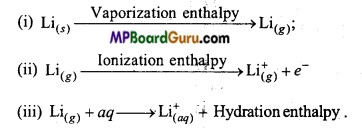Due to small size of their ions, hydration enthalpy of Lithium is maximum. Though, ionization enthalpy of Li is maximum among Alkali metals but its hydration enthalpy is more than ionization enthalpy. Thus, due to high hydration enthalpy, Lithium is strongly reducing in aqueous solution.

Question 5.
Why are Alkali metals and Alkaline earth metals not obtained by chemical reduction methods? Explain.
Answer:

• Alkali and Alkaline earth metals are itself strong reducing agents. Thus, these metals cannot be obtained by chemical reduction methods of their oxides and other compounds.
• These metals are highly electropositive in nature: Therefore, these cannot be displaced from aqueous solution of their salts by other metals.

Question 6.
What is the reason that alkali metals form M+ ion and not M2+ ion?
Answer:
Alkali metals consist of one electron in the outermost shell which it can loose easily and achieve stable inert gas configuration. They do not possess the tendency to form M2+ type of ion because the value of ionization energy necessary to remove the second electron is very high.Question 7.
Which of the alkali metal is strongly reducing and why?
Answer:
Reducing tendency of an element depends on its standard electrode potential. Elements whose standard electrode potentials are negative behave as reducing agents and higher the negative value of the standard electrode potentials of an element, stronger is its reducing tendency. Lithium has the highest negative standard electrode potential, therefore, it is strongly reducing.

Question 8.
Alkali metals do not exist in free state in nature. Why? Or, Alkali metals always form ionic compounds. Why?
Answer:
Due to the large size of alkali metals, their ionization energy is less, therefore it can easily lose electron and form cation. Therefore, being strongly electropositive and extremely reactive it easily reacts with electronegative elements like: O, Moisture, CO2 etc. present in the atmosphere and forms ionic compounds. Therefore, they do not exist in free state in nature.

Question 9.
Why do alkali metals give flame test?
Answer:
Alkali metals give their characteristic colour to the oxidizing flame. The valence electron gets excited by absorbing energy from the flame. On falling back from higher energy state to lower energy state it emits radiations of lower energy of the range of visible region. Different alkali metals give different colour because they are having different ionization energy. The alkali metals which have high I.E. will emit light having minimum frequency and vice-versa.

Question 10.
Why Be and Mg do not give flame test?
Answer:
Except beryllium and magnesium all the alkaline earth metals impart characteristic colours to Bunsen flame.
Due to small size of Be and Mg atoms, the energy required to excite the valence electrons is very high which is not obtained in Bunsen flame. That is why Be and Mg do not impart any colour to flame.

Question 11.
When an alkali metal is dissolved in liquid ammonia then the solution obtains various colours. State the cause of such type of colour.
Answer:
The colour of dilute solution of alkali metal in liquid ammonia is dark blue because ammoniated electrons absorb energy from visible range of light.
M + (x + y)NH3→ [M(NH3)x]+ +[e(NH3)y]Ammoniated electron
If concentration of solution is increased, it gets converted to bronze colour.

Question 12.
State two-two use of each of the following :
(a) Caustic soda,
(b) Sodium carbonate,
(c) Quick lime.
Answer:
(a) Caustic soda :

• It is used in the preparation of soap, paper, artificial silk etc.
• In the textile industry, it is used for mercerising cotton fabrics.

(b) Sodium carbonate :

• It is used in softening of water and washing.
• It is used in the manufacture of glass, soap, borax, caustic soda etc.

(c) Quick lime:

• It is used in the preparation of washing soda from caustic soda. ,
• It is used in the purification of sugar and in the manufacture of dyestuffs.

Question 13.
Alkali metals form blue coloured solution on dissolving in ammonia, which is good conductor of electricity? Explain, why?
Answer:
Alkali metals dissolve in liquor ammonia to form deep blue coloured solution of high electrical conductivity.
M + (x +y)NH3 → [M(NH3)x]+ +[e (NH3)y].
The blue colour of the solution is due to ammoniated electrons. Positive ion and electrons are responsible for the conductivity.Question 14.
Na is alkaline or Na2O. Explain.
Answer:
Na2O is alkaline because it reacts with water to form NaOH. Na also reacts with water to form NaOH but it forms Na2O firstly and then NaOH. Thus, Na2O is alkaline.
4Na + 2H2O → 2Na2O + 2H2
Na2O + H2O → 2NaOH

Question 15.
Differentiate Alkali metals and Alkaline earth metals on the basis of following points: ‘
(i) Reaction with nitrogen.
(ii) Effect of heat on carbonates.
(iii) Solubility of sulphates in water.
Answer:
Differences of Alkali metals and Alkaline earth metals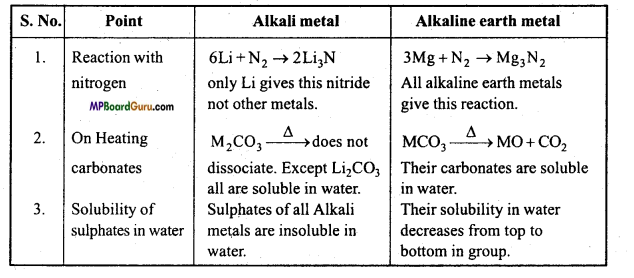Question 16.
Draw the structure of the following :
(i) BeCl2 (vapour),
(ii) BeCl2 (solid).
Answer:
(i) In vapour state, it exists as a bridged chloride dimer.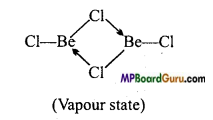(ii) In solid state, it forms a polymeric chain with chlorine bond.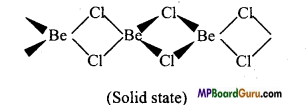Question 17.
Hydroxides and carbonates of sodium and potassium are soluble in water, whereas corresponding salts of magnesium and calcium are partially soluble. Explain.
Answer:
Solubility of a salt in water depends on its Hydration energy and Lattice energy.
ΔHsolution = ΔHHyderation energy +ΔHLattice energy

Higher the negative value of ΔH Solution, lesser is the solubility of the compound. Hydration energy of hydroxides and carbonates of sodium and potassium is higher than their Lattice energy. Thus, they are soluble in water.
Lattice energy of magnesium and calcium hydroxides is more than their hydration energy. Thus, they are partially soluble in water.

Question 18.
Salts of Lithium are generally hydrated, whereas salts of other alkali metals are generally anhydrous. Why ?
Answer:
Due to smallest size among alkali metals, hydration energy of Li+ is least. That is why lithium salts are generally hydrated and salts of other alkali metals are normally anhydrous.Question 19.
LiF is nearly soluble in water, whereas LiCl is not only soluble in water but is also soluble in acetone. Give reason.
Ans. LiF, due to high lattice energy is nearly soluble in water, but due to high hydration energy of Li ions, LiCl is also soluble in water. But due to specific covalent character of LiCl, it is also soluble in acetone (Since covalent character increases with increase in size of anion. Thus, order of solubility is :
LiF < LiCl < LiBr < Lil

Question 20.
Alkali metals lose their metallic lustre, when kept in open air. Why?
Answer:
All metals possess a specific lustre known as metallic lustre. Due to large size of alkali metals, their ionization energy is very less. Therefore, they are highly reactive and strongly electropositive and react with moisture, O2 and CO2 present in the atmosphere and form basic carbonate. Due to formation of oxide and carbonate layer on their surface, they lose their metallic lustre.Question 21.
Differentiate between Alkali metals and Alkaline earth metals.
Answer:
Differences between Alkali metals and Alkaline earth metals

 Alkali metal Alkaline earth metal 1. They show +1 valency. They show +2 valency. 2. Their hydroxides are strong alkalines. Their hydroxides are comparatively weak alkalines. 3. Their carbonates, sulphates and phosphate are soluble in water. These compounds of alkaline earth metals are insoluble in water. 4. Magnitude of their ionization energy is comparatively low. Magnitude of their ionization energy is high. 5. These are lustrous, malleable and ductile. These are comparatively less.

Question 22.
Among LiCl and RbCl, which is strongly ionic and why?
Answer:
RbCl is stronger ionic than LiCl because due to small size of Li, its ionization energy is very high. Therefore, it forms covalent compounds, whereas due to larger size of Rb, its ionization energy is very less, due to which it easily loses electron and forms cation. Therefore, its compounds show strong ionic character.

Question 23.
Among alkali metals and Alkaline earth metals, whose carbonate is soluble in water and why ? Or, Carbonates of Alkali metals are soluble in water whereas carbonates of Alkaline earth metals are insoluble in water. Why?
Answer:
Carbonates of Alkali metals are soluble in water because their hydration energy is more than their lattice energy, whereas due to small size and high charge density of Alkaline earth metals, their lattice energy is high and is more than their hydration energy. Therefore, carbonates of Alkaline earth metals are insoluble in water.

Question 24.
Explain the importance of sodium,potassium, magnesium and calcium in biological fluids.
Answer:
Sodium and potassium ions play an important role in interstitial fluids. These ions participate in the transmission of nerve signals. Potassium ions are the most abundant cations within cell fluids, where they activate many enzymes, participate in the oxidation of glucose to produce ATP.

The main pigment for the absorption of light in plants is chlorophyll which contains magnesium.
About 99% of body calcium is present in bones and teeth. It also plays important roles in neuromuscular function, intemeuronal transmission, cell membrane integrity and blood coagulation.

Question 25.
Though value of second ionization energy of Alkaline earth metals is more than their first ionisation energy still these metals show +2 oxidation state and not +l. Why?
Answer:
The value of second ionization energy of Alkaline earth metal is more than its first ionization energy still their maximum compounds show +2 oxidation state due to the following reasons:

• Electronic configuration of bivalent ion is similar to inert gases.
• During the formation of bivalent ion they release large amount of hydration energy which compensates the high value of second ionization energy.
• Due to small size of M2+ ion their hydration energy is high which compensate the high value of second ionization energy.

Question 26.
State the dissimilarities in BeCl2 and other alkaline earth metal chlorides.
Answer:

1. BeCl2 is a covalent compound whereas chlorides of other alkaline earth metals are ionic.
2. BeCl2 is insoluble in water whereas other chlorides of other alkaline earth metals are soluble in water.
3. BeCl2 is soluble in organic solvents whereas chlorides of other alkaline earth metals are soluble in organic solvents.
4. Melting and boiling points of BeCl2 is low whereas that of chlorides of other alkaline earth metals is high.

Question 27.
Solubility of BaS04 is less than CaSO4. Why?
Answer:
Solubility of sulphates of alkaline earth metals decreases on moving down the group because though lattice energy nearly remains same, their hydration energy decreases down the group due in increase in atomic radius. Value of hydration energy goes on becoming less than lattice energy due to which solubility decreases.

Question 28.
Ionization energy of Be is more than B. Why?
Answer: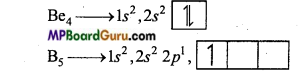In Be, s-orbital is complete whereas in B,p -orbital is incomplete. Half filled or fully filled orbital is more stable than incomplete orbital and huge amount of energy is required to remove the electron from them. Therefore, ionization energy of Be is more than Boron.

Question 29.
How is sodium carbonate prepared by ammonia soda process? Write its principle.
Answer:
In this process, first concentration NaCl solution is saturated with NH3 by which ammonical sodium chloride is formed.
CO2 gas is then passed through this ammonical sodium chloride solution when ammonium bicarbonate is formed which reacts with sodium chloride to form sodium bicarbonate.
NH3 + CO2 + H2O → NH4HCO3
NH4HCO3 + NaCl → NaHCO3 + NH4Cl
Sodium bicarbonate being partially soluble settles at the bottom as precipitate. It is filtered and ignited to form sodium carbonate.
2NaHCO3→ Na2CO3 + H2O + CO2.

Question 30.
How are (1) sodium bicarbonate (2) sodium hydroxide and (3) sodium silicate obtained from sodium carbonate?
Answer:
1. On passing CO2 gas through aqueous sodium carbonate solution, white precipitate of sodium bicarbonate is obtained.
Na2CO3 + H2O + CO2 → 2NaHCO3
2. On boiling sodium carbonate with lime water, sodium hydroxide is formed.
Na2CO3 + Ca(OH)2 → 2NaOH + CaCO3
3. On heating sodium carbonate with silica, sodium silicate is formed.
Na2CO3 + SiO2 →Na2SiO3 + CO2.

Question 31.
What is baking soda? Write its method of preparation, properties and use.
Answer:
Definition: Sodium hydrogen carbonate is known as Baking soda and its formula is NaHCO3.
Method of preparation :
1. In Ammonia Soda process, sodium bicarbonate is obtained as an intermediate compound.
NH4HCO3 + NaCl →NaHCO3 + NH4Cl

2. Sodium bicarbonate is formed by passing C02 gas through sodium carbonate solu¬tion.
Na2CO3 + H2O + CO2 →2NaHCO3
Properties: It is a white crystalline substance, partially soluble in water. Its aqueous solution is weakly basic.

Uses :

• In the preparation of baking powder,
• As a medicine to reduce acidity of the stomach,
• In fire extinguishers.

Question 32.
Describe briefly Le-Blanc process of preparation of sodium carbonate and explain the cause of superiority of Solvay process over Le-Blanc process.
Answer:
Le-Blanc process: The names of the three steps for the formation of sodium carbonate are as follows :
(i) Preparation of salt cake: Sodium chloride when heated with cone. H2SO4 produces sodium sulphate (salt lake) and HCl gas.
(ii) Black ash: On heating the mixture of sodium sulphate, calcium carbonate and coke gives a mixture of sodium carbonate and calcium sulphide which is known as Black ash.

(iii) Production of black ash from common salt: Mixture is mixed with water and filtered. Sodium carbonate is soluble in water, therefore it remains infiltrate, CaS is removed as residue. The evaporation of filtrate gives solid sodium carbonate..
2 NaCl + H2SO4 →Na2SO4 + 2HCl
Na2SO4 + 4C → Na2s + 4CO
Na2S + CaCO3 → (Na2CO3+CaS) Black ash

Superiority of Solvay process over Le-Blanc process :

• Solvay process is cheap.
• Pure Na2CO3 is formed in Solvay process.
• No chemical fumes are released in Solvay process,
• In Solvay process, NaHCO3 is formed in the middle which is a useful compound.Question 33.
Describe Hargreave-Bird cell of sodium carbonate.
Answer:
Hargreave-Bird cell contains a carbon anode and a porous copper cathode which are separated by an asbestos sheet. By the electrolysis of NaCl solution, sodium and chlorine are formed. In the cell, steam and CO2 are passed at the outer side of asbestos, which reacts with Na to form Na2CO3. On evaporating this solution Na2CO3.10H2O is obtained.

2 NaCl ⇌ 2Na++2Cl
2Na++2e → 2Na
At cathode: 2Na + 2H2O →2NaOH + H2
2NaOH + CO2 →Na2CO3 + H2O
At anode: 2Cl – 2e → 2Cl
Cl + Cl → Cl2.

Question 34.
Explain Nelson cell process of preparation of sodium hydroxide with diagram.
Answer:
Nelson cell consists of a cylindrical asbestos sheet covered by porous steel lining arranged inside a steel tank. The porous steel lining acts as cathode. NaCl solution is filled in this chamber. Carbon rod acts as anode.
On electrolysis, sodium ions are released which pass the asbestos sheet and after releasing at cathode react with the steam entering the tank and form NaOH.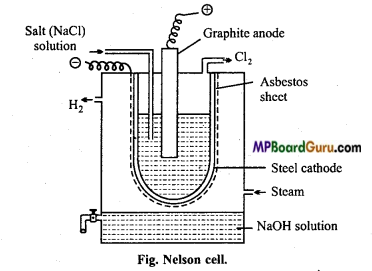2NaCl ⇌ 2Na++2Cl
At cathode : 2Na+ +2e → 2Na
2Na + 2H2O →2NaOH + H2
At anode : 2Cl – 2e → 2Cl
Cl + Cl → Cl2.

Question 35.
Write method of preparation, properties and uses of slaked lime.
Answer:
Method of preparation :
1. On sprinkling water on quick lime, slaked lime is obtained.
CaO + H2O → Ca(OH)2
2. Ca(OH)2 is obtained by the reaction of a base on calcium salt.
Ca(NO3)2 + 2 NaOH → Ca(OH)2 + 2NaNO3

Properties :
1. On heating it to 400°C, calcium oxide is formed.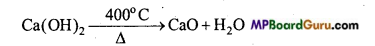2. On passing CO2 through lime water, milky white precipitate of CaCO3 is formed.
Ca(OH)2 +CO2 → CaCO3 + H2O
3. On passing CO2 through lime water for a longer time, milky white colour disappears.
CaCO3 + CO2 + H2O → Ca(HCO3)2
4. On passing Cl2 gas through dry slaked lime, bleaching powder is obtained.
Ca(OH)2 + Cl2 → CaOCl2 + H2O

Uses :

• In the manufacture of caustic soda and bleaching powder.
• In the purification of coal gas.
• In whitewashing.
• In the manufacture of ammonia.

Question 36.
Describe the soda-lime method for preparation of sodium hydroxide with chemical equation.
Answer:
Soda-lime method is also known as causticizing process. In this method, sodium hydroxide is obtained by boiling sodium carbonate solution with slaked lime.
Na2CO3 + Ca(OH)2 ⇌ 2NaOH + CaCO3
This reaction is reversible but due to precipitation of calcium carbonate, the reaction proceeds only in forward direction.

A mixture of sodium carbonate and slaked lime is taken in iron tanks. The mixture is heated to 80-85°C by steam. Precipitated calcium carbonate is separated periodically. The filterate is treated with dilute hydrochloric acid for testing the presence of sodium carbonate. More lime is added when effervescence occurs. Dilute hydrochloric acid is added when no more effervescence occurs and the solution is filtered to separate precipitate of calcium carbonate. Filterate obtained on evaporation in iron containers give 98% pure sodium hydroxide.

The s-Block Elements Class 11 Important Questions Long Answer Type

Question 1.
Write notes on each of the following observations :
(a) Mobility of alkali metal ions in aqueous solution are :
Li+ < Na + < K+ < Rb+ < Cs+
(b) Lithium is the only alkali metal which forms nitride.
(c) E° for M2+(aq) + 2e → M(s) (Where M = Ca, Sr or Ba) is nearly constant.
Answer:
(a) Smaller the size of an ion, higher is its hydration and higher the hydration of an ion lower is its mobility. Thus, order of hydration ability is as follows: ‘
Li+ < Na + < K+ < Rb+ < Cs+
Thus, ionic mobility is in the opposite order.

(b) Due to its small size, among alkali metals only Lithium forms nitride.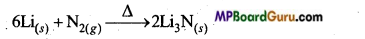(c) E° for M2+(aq) + 2e → M(s) where M = Ca, Sr or Ba, value of E° is nearly same.

For a M2+/M electrode, value of E° depends on three factors:

• Enthalpy of Vaporization,
• Ionization enthalpy,
• Hydration enthalpy.
Since, the combined effect of all these three factors is nearly same for Ca, Sr and Ba, therefore value of their electrode potentials is also nearly same.

Question 2.
How is lithium different from other members of its group?
Answer:
Anomalous behaviour of lithium: Properties of lithium are different than other members of the group due to the given reasons :
(i) Size of lithium atom and ion is very small.
(ii) Due to small size, polarizing power of lithium-ion is high, due to which compounds possess covalent character.
(iii) In comparison to other alkali metals its electropositive nature is less and ionization energy is high.
Lithium differ from alkali metals of group I due to following properties :
(i) Lithium is comparatively harder than the other alkali metals.
(ii) Melting and boiling point pf lithium is comparatively high.
(iii) Lithium reacts with oxygen to form only normal oxide (Li2O), whereas other metals form peroxide (M2O2) and superoxide (MO2).
(iv) Lithium hydride (LiH) is more stable as compared to hydrides of other metals.
(v) Lithium hydroxide (LiOH) is a weak base and is partially soluble in water, but hydroxides of other metals of the group are more soluble in water.
(vi) Lithium forms nitride (Li3N) with nitrogen, whereas other metals of the group do not form nitride.
(vii) Lithium nitrate when heated decomposes to nitrogen dioxide and oxygen.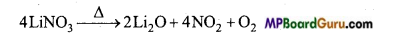Whereas sodium nitrate and potassium nitrate when heated strongly form correspond¬ing nitrate with the release of oxygen.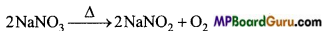(viii) Lithium carbonate and lithium hydroxide decompose on heating strongly, but carbonates and hydroxides of other metals do not decompose on heating.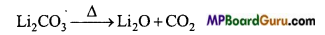(ix) Fluoride, phosphate, oxalate and carbonate of Lithium are partially soluble in water, whereas corresponding salts of other metals are soluble in water.Question 3.
What is diagonal relationship? Write diagonal relationship between Li and Mg.
Answer:
Diagonal relationship: Some elements of second period show similarity with some elements of third period diagonally.
This is known as diagonal relationship. Diagonal relationship between Li and Mg :

• Atomic radius of Lithium is 1.34 Å and atomic radius of Mg is 1.36 Å.
• Polarizing power of Li+ and Mg2+ is nearly same.
• Li and Mg both are hard.
• Electronegativity of Li and Mg is (1.0 and 1.2) same.
• Melting and boiling point of Li and Mg is high.
• Both Li and Mg react with N2 to form nitride.
• Both Li and Mg react with oxygen to form monoxide.
• Li and Mg both dissociate water to give H2.
• Carbonates of both Li and Mg give CO2 on heating.
• Both LiOH and Mg(OH)2 are weak bases.

Question 4.
What are similarities between Alkali metal and Alkaline earth metals?
Answer:

• Alkali metals and Alkaline earth metals are not obtained in free state in nature.
• Oxides of alkali metals and alkaline earth metals dissolve in water to form strong bases.
• Alkali metals and Alkaline earth metals are soft and bright.
• On keeping in air, their surface become dull.
• Alkali metals and Alkaline earth metals (except Be and Mg) give flame test.
• Alkali metals and Alkaline earth metals are strong reducing agents.
• Alkali metals and Alkaline earth metals both form ionic compounds.
• Nitrate and halide of both are soluble in water.

Question 5.
Be shows anomalous behaviour as compared to other members of its group. Explain.
Answer:
Anomalous behaviour of beryllium: Being the first member of family, beryllium shows anomalous behaviour as compared to other members of family. It is because of following reasons:

• Smallest size of the atom and the ion.
• High ionization energy, electronegativity and heat of hydration.
• Vacant d-orbitals are not present.

Beryllium shows the following different properties from other members of the group :
1. Beryllium is hard, whereas other metals are soft.

• Beryllium does not liberate hydrogen quickly from acids whereas other metals liberate quickly.
• Beryllium carbide form methane by reaction with water whereas other members give acetylene.
Be2C + 2H2O → 2BeO + CH4
CaC2 +2H2O → Ca(OH)2 + C2H2
• Beryllium oxide is amphoteric whereas oxides of other metals of the group are basic.
• Beryllium and magnesium do not impart any colour to the flame whereas all other members impart colour.
• Beryllium react with hydrogen slowly whereas others react quickly.
• Compounds of beryllium are generally covalent where other members of the group form ionic compounds.

Question 6.
Explain why :
(a) Solution of Na2CO3 is alkaline.
(b) Alkali metals are obtained by the electrolysis of their corresponding fused chlorides.
(c) Sodium is more useful than Potassium.
Answer:
(a) Na2CO3 is a salt of a weak acid (H2CO3) and a strong base (NaOH). Thus, on hydrolysis it gives base (NaOH) due to which its aqueous solution is alkaline.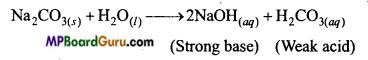(b)
1. Alkali metals are strongly reducing, thus they cannot be extracted by their oxides and compounds.
2. Due to high electropositive nature, these cannot be displaced by other metals from their salt solutions.
3. Alkali metals cannot be obtained by the electrolysis of aqueous solution of their salts because at cathode in place of Na metal H2 is liberated. Due to this, Alkali metals are obtained by the electrolysis of their molten chlorides.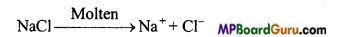At Cathode:2Cl → Cl2 + 2e
At Anode : 2Na+ + 2e → 2Na

(c) As compared to potassium, sodium is more important because it is reactive but not very reactive as potassium. Other uses of sodium are :

• As a coolant in nuclear plants.
• In the manufacture of Tetraethyl lead (TEL) used as antiknock agent for petrol.
4C2H5Cl + 4Na- Pb → (C2H5)4 Pb + 3Pb+4NaCl (TEL).

Question 7.
Explain diagonal relationship between Be and Al.
Answer:
Diagonal relationship between Beryllium and Aluminium: Beryllium resembles aluminium of group 3 in the following properties :

• Both beryllium and aluminium form covalent compounds.
• Both metals are passive towards reaction with concentrated HNO3, because they are covered with a layer of oxide on their surface.
• Both the metals are of weak electropositive nature.
• Both do not form hydride quickly.
• Carbides of both the metals react with water to form methane.
Be2C + 2H2O → 2BeO + CH4
Al4C3 + 6H2O → 2 Al2O3 + 3CH4
• Oxides of both are soluble in water and form hydroxides which are amphoteric in nature and react with acid and base to form salt.
BeO + 2 HCl → BeCl2 + H2O
Al2O3 +6HCl → 2AlCl3 +3H2O
BeO + 2NaOH >Na2Be02 + H2O
Al2O3 + 2NaOH → 2NaAlO2 + H2O
• Both Be and Al do not give colour to the flame.

Question 8.
How is sodium carbonate manufactured by Solvay process?
Answer:
Principle: In this process, first concentrated solution of sodium chloride (Brine) is saturated with NH3 to form ammonical sodium chloride. On passing CO2 gas to this, ammonium bicarbonate is formed which reacts with sodium chloride and forms sodium bicarbonate. Precipitate of sodium bicarbonate is filtered which on calcination gives sodium carbonate.’
NH3+CO2+H2O → NH4HCO3
NH4HCO3 + NaCl →NaHCO3 +NH4Cl
2NaHCO3 → Na2CO3 + H2O + CO2

CO2 formed is used again for carbonation NH4Cl formed on treatment with slaked lime gives NH3 which is used for saturation of brine.
2NH4Cl + Ca(OH)2 →CaCl2 +2H2O + 2NH3
The various steps and reactions involved are given below :

Saturating tank: In this tank brine (NaCl) is saturated with ammonia. NaCl solution is introduced from the top while ammonia is introduced from bottom so that ammonium brine is formed and which collects at bottom. Calcium and magnesium salts are present as impurity in sodium chloride solution. They get precipitated as hydroxides by ammonium hydroxide.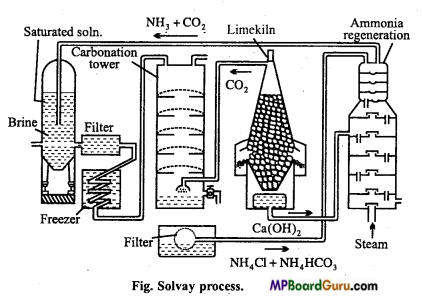Filter: Ammonical brine is filtered to removed the precipitated hydroxides of calcium and magnesium.

Cooler: The filtrate is cooled by passing through condenser. Cooling is necessary because when ammonia dissolves in brine a lot of heat is produced.

Carbonating tower: The cold solution of brine saturated with ammonia is intro¬duced into the carbonating tank from top.
Carbonating tower is fitted with a number of compound diaphragms each made of a horizontal iron plate.

Following reactions take place here :
2NH3 + CO2 +H2O → (NH4)2CO3
(NH4)2CO3 +2NaCl → Na2CO3 + 2NH4Cl
Na2CO3 +H2O + CO2 → 2NaHCO3

Vacuum filter: The precipitated sodium bicarbonate along with solution of traces of ammonium carbonate and ammonium chloride is passed through rotatory vacuum filter at the bottom of tower where socfium bicarbonate separates leaving behind mother liquor containing ammonium chloride.

Limekiln: Here limestone is burnt to produce quick lime and carbon dioxide.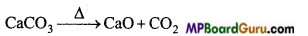Formed CaO reacts with water to form slaked lime.
CaO + H2O →Ca(OH)2

Ammonia recovery tower: The mother liquor obtained from rotatory filter pump containing ammonium chloride is introduced into the ammonia recovery tank from the top part while the milk of lime is introduced from the top of lower part and steam is introduced into the tower from the bottom, ammonium chloride reacts with milk of lime to produce ammonia.
2NH4Cl + Ca(OH)2 → 2NH3 +CaCl2 +2H2O

Sodium bicarbonate obtained from rotatory filters is ignited in a specially constructed cylindrical vessels when sodium carbonate is formed and CO2 evolved is collected and used again.
2NaHCO3 →Na2CO3 + H2O+CO2.Question 9.
When a metal of group-1 is dissolved in liquid ammonia the following observations are obtained : ,
(a) Initially blue solution is obtained.
(b) On concentrating the solution, blue colour gets converted to bronze. Discuss the blue colour of the solution. Write the name of the product formed on keeping the solution for some time.
Answer:
(a) On dissolving the metals of group-1 in liquid ammonia the following reaction is obtained:
The blue colour of the solution is due to ammoniated electrons which absorbs the corresponding energy of the visible range and provides blue colour to the solution.
M+(x+y)NH3 → [M(NH3)x]+ + [e(NH3)y]

(b) In concentrated solution, due to formation of metal ion layer the blue colour gets converted to bronze colour. Blue colour releases hydrogen on being kept for some time and amide is formed.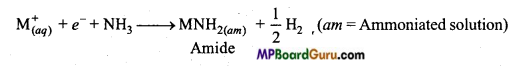Question 10.
Stability of peroxides and superoxides of Alkali metals decreases on moving down the group. Discuss with example.
Answer:
Stability of peroxides and superoxides of metal ion increases with increase in size.
Thus, KO2 < RbO2 < CsO2
Cause of formation of various oxide by alkali metals with oxygen is the formation of a boundary of strong electropositive region around the metal cation. Size of Li+ is the smallest due to which it does not allow O2- ion to react with O2. Size of Na+ is bigger than Li thusn its positivity decreases by Li+ region. Bigger ions like K+, Rb+ and Cs+ allow O2-2 ion again to react with O2 to form superoxide (O2)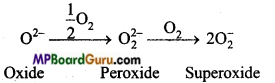With the increase in size of metal ions, stability of peroxides and superoxides also increases. Its main cause is that due to lattice energy effect, larger anion and larger cation provide stability.

Question 11.
Explain Castner-Kellner cell with labelled diagram to obtain sodium hydroxide.
Answer:
It consists of a rectangular iron tank which is divided into three parts by state partitions. These partitions do not touch the bottom of the tank which is covered by mercury. Layers of mercury are separated from each other in all the three parts. Mercuiy layer can move here and there by mechanical arrangement. NaOH solution is filled in the outer compartment in which graphite anode is immersed. In the middle dilute NaOH solution is filled in which iron cathode is immersed. On passing electric current, mercury in middle compartment acts as anode and in the outer compartments act as cathode by induction. Cl2 is released in the outer compartment at anode.

Sodium released at cathode reacts with mercury to form sodium amalgam. Sodium amalgam is introduced in the central compartment by wheels. Here sodium amalgam acts as anode and iron rod acts as cathode. In this portion NaOH is filled. On passing electric current OH ions are released at anode and reacts with sodium present in amalgam to form NaOH and H2 gas is released.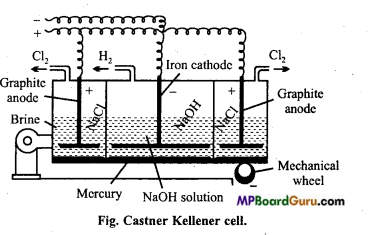Reaction : In outer compartment:
2NaCl ⇌ 2Na+ +2Cl
At cathode:
2Na+ +2e → 2Na
2Na + xHg → HgxNa2
At anode:
2Cl – 2e →2Cl
Cl + Cl → Cl2

In middle compartment:
NaOH ⇌ Na+ + OH
At cathode:
2NaHg + 2H2O → 2NaOH+2Hg + H2
Na+ +e →Na
2Na + 2H2O →2NaOH + H2
At anode:
2OH → 2OH + 2e
Hgx Na2 +2OH → 2NaOH+xHg.

Question 12.
When water is mixed in a compound of calcium
(A) solution of compound
(B) is formed. On passing CO2 through this solution, due to the formation of compound
(C) it turns milky. On passing excess of carbon dioxide, compound
(D) is formed and due to this milky colour disappears. Identify the compound (A), (B), (C) and (D) and explain the cause of disappearance of milky colour in the last step.
Answer:
On passing CO2 in the solution of compound
(B), the appearance of milky colour indicates that compound (B) is slaked lime [Ca(OH)2] and compound
(C) is calcium carbonate. Since compound (B) is formed on adding H2O to compound (A), thus compound A is quick lime (CaO).
This reactions are as Follows: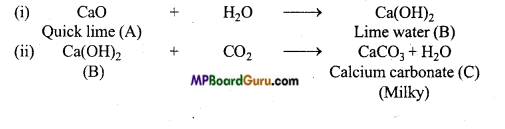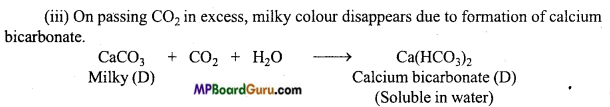Question 13.
Write method of preparation of calcium oxide, chemical properties and its uses.
Answer:
Calcium oxide is formed by heating lime stone.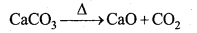The reaction is reversible, thus it is necessary to remove CO2. Temperature of the reaction should be 900°C because at high temperature by the reaction of sand and lime fusible silicate is formed.
Coal is burnt at the side of the furnace. Lime stone is added slowly from the top, which dissociates on coming down. CO2 gas releases from the upper part.
It is liquefied and stored in the cylinders.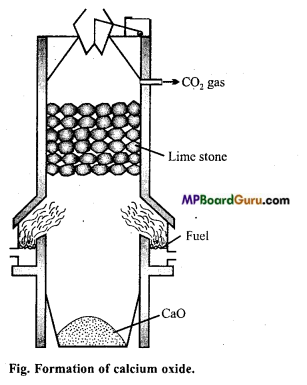Chemical properties :
1. It absorbs moist air andCO2 to form Ca(OH)2 andCaCO3.
CaO+ H2O → Ca(OH)2
CaO+ H2O → CaCO,
2. It is a strong basic oxide which reacts with acids to form salts.
CaO + 2HCl → CaCl2 + H2O
CaO + SiO2 → CaSiO3
3. On heating with ammonium salts NH3 gas is formed.
2NH4Cl + CaO → CaCl2 +H2O + 2NH3
4. On heating carbon and calcium oxide CaC2 is obtained.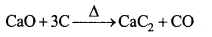Uses :

• As a flux in metallurgy,
• In drying of alcohols and gases,
• For producing lime light,
• In the purification of coal gas,
• In making paper.

Question 14.
What happens when :
(1) On reacting NaOH with Zn or Al.
(2) On reacting NaOH with S.
(3) On reacting NaOH with halogen.
(4) On reacting NaOH with metal oxide.
(5) On reacting NaOH with metal salts.
Answer:
1. On reacting NaOH with Zn or Al, complex salt is obtained and hydrogen gas is released.
Zn + 2NaOH →Na2ZnO2 + H2
2Al + 2 NaOH + 2H2O → 2NaAlO2 + 3H2

2. On reacting NaOH with sulphur, hypo is formed.
4S + 6NaOH → Na2S2O3 + 2Na2S + 3H2O
8S + 2Na2S → 2Na2S5

3. Reaction with halogen :
(a) On reacting dilute and cold NaOH with chlorine, sodium hypochloride is formed.
2NaOH +Cl2 → NaCl + NaClO+ H2O
(b) On reacting hot and cone. NaOH with chlorine, sodium chlorate is obtained.
6NaOH + 3Cl2 → 5NaCl+NaClO3 + 3H2O

4. On reacting NaOH with metal oxide, complex salt is obtained.
ZnO + 2NaOH → Na2ZnO2+H2O

5. On reacting NaOH with metallic salts, precipitate of metal hydroxides are obtained.
CuSO4 + 2NaOH → Na2SO4 + Cu(OH)2
3NaOH + FeCl3 → Fe(OH)3 + 3NaCl
2NaOH + 2 AgNO3 → Ag2O + 2NaNO3 + H2OQuestion 15.
State the importance of:
(a) Lime stone,
(b) Cement,
(c) Plaster of Paris.
Answer:
(a) Lime stone CaCO3:

• With magnesium carbonate, it is used as a flux in the extraction of metals like iron.
• It is used as an antacid, mild abrasive in toothpaste and a filler in cosmetics.

(b) Cement:

• It is an important compound for the construction of buildings.
• It is used in concrete and reinforced concrete, in plastering and in the construction ofbuildings.

(c) Plaster of Paris :

• It is used in building industry as well as plasters in bone fracture.
• It is also used in dentistry, in ornamental work and for making casts and statues and busts.

Question 16.
Write short notes on :
(i) Plaster of Paris and
(ii) Lime.
Answer:
(i) Plaster of Paris : It is formed from gypsum by heating up to 120-130°C in absence of organic fuel.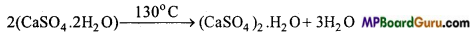On adding water it again change into gypsum and becomes hard.
(CaSO4)2.H2O + 3H2O → 2CaSO4.2H2O

Uses :
(a) It is used in plasters in bone fracture.
(b) In preparation of toys, decorative items and in fireproof bricks.

(ii) Lime : Calcium oxide and hydroxide is known as lime.
(A) Quick lime (CaO): It is prepared by heating limestone. .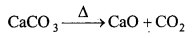Properties : (a) It forms limelight on heating with oxygen.
(b) Reaction with air and moisture.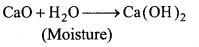CaO + CO2 → CaCO3.

Uses :
(a) As a flux (basic) in metallurgy.
(b) In producing limelight.

Question 17.
Discuss the various reactions that occur in Solvay process.
Answer:
In Solvay process, CO2 is passed through brine (cone. NaCl so In.) saturated with ammonia when easily soluble sodium bicarbonate is formed.
NaCl + NH3 + CO2 + H2O → NaHCO3 ↓ + NH4C1
The sodium bicarbonate formed is filtered, dried and heated to form sodium carbonate.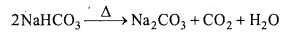CO2 used in the carbonating tower is obtained by heating calcium carbonate. CaO formed in this process is dissolved in water to form slaked lime.Ammonia is regenerated in the regeneration tower by heating NH4Cl and Ca(OH)2.
2NH4Cl + Ca(OH)2→ 2NH3 + CaCl2 + 2H2O

Question 18.
What are the common physical and chemical characteristics of Alkali metals ?
Answer:
Physical properties :
(i) Alkali metals are silvery white, soft and tight metals.
(ii) These have low density (due to large size). It increases on moving down the group. Though potassium is lighter than sodium.
(iii) Alkali metals have low melting and boiling points. Because due to presence of only one valence electron, weak metallic bond is present between them.
(iv) Alkali metals and their salts give characteristic colour to the flame, because their valence electrons easily get excited from lower energy level to higher energy level.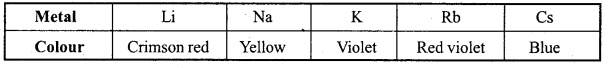Chemical properties : Alkali metals are highly reactive due to their low ionization enthalpy. Their reactivity increases down the group.
(i) Reaction with air : Alkali metals form oxide in presence of air and become dull. They form lithium monoxide, sodium peroxide and others form superoxide.
4Li + O2 → 2Li2O (Monoxide)
2Na + O2 → Na2O2 (Peroxide)
M + O2 → MO2 (Superoxide) (M = K, Rb, Cs)
Lithium reacts directly with nitrogen of the air to form nitride (Li3N).

(ii) Reaction with water : Alkali metals react with water to form hydroxides and dihydrogen.
2M + 2H2O → 2M+ + 2OH + H2 (M = Alkali metal)
Reaction of Li with water is less than that of sodium.
Alkali metals also react with proton donor species like alcohol, alkyne and gaseous ammonia.

(iii) Reaction with dihydrogen : Alkali metals react with dihydrogen to form ionic hydride.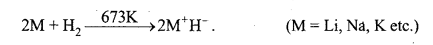(iv) Reaction with halogen : Alkali metals easily react with halogens to form ionic halides.
2M + X2 → 2MX Metal halide . (M = Alkali metal).
(v) Reducing nature : Alkali metals easily lose their valence electrons, thus they are strongly reducing.
Na → Na+ + e
Lithium is strongly reducing whereas sodium is least reducing.
(vi) Solubility in liquid ammonia : All alkali metals dissolve in liquid ammonia to form deep blue solution.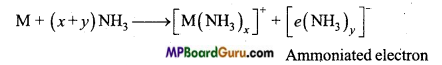(vii) All alkali metals react with liquid ammonia to form alloys. With mercury they form amalgams and the reactions are exothermic.

Question 19.
Discuss the general characteristics and gradation in properties of Alkaline earth metals.
Answer:
Elements of group-2 Be, Mg, Ca, Sr, Ba and Ca and together known as Alkaline earth metals (except Be).
General characteristics :
[A] Atomic properties :
(i) Electronic configuration : Their general electronic configuration is represented as [Noble gas]ns2.
(ii) Atomic and ionic radius : Their atomic and ionic radii are smaller than the nearest
alkali metals of the same period. Atomic and ionic radii in the group increases with the increase in atomic number. ‘
(iii) Ionization enthalpy : First ionization energy of alkaline earth metals due to their smaller size is more than that of alkali metals[ionization enthalpy ∝ $$\frac{1}{\text { Atomic size }}$$ ionization enthalpy of Alkaline earth metals is less than the corresponding alkali metals.

(iv) Hydration enthalpy of Alkaline earth metals like alkali metal ions decreases gradually on moving down the group.
Be2+ > Mg2+ > Ca2+ > Sr2+ > Ba2+
Hydration enthalpy of Alkaline earth metals is less than the corresponding Alkali metal ions.

[B] Physical properties : (i) These metals are silvery white, lustrous and soft (but harder than alkali metals).
(ii) The melting and boiling of these metals are higher than the corresponding alkali metals due to their smaller size. The trend is however not systematic.
(iii) Flame colouration : Be and Mg do not impart any colour to the flame. The various colours is due to difference in energy required for the excitation of electrons.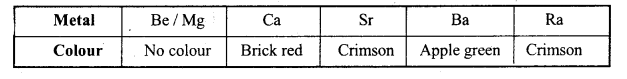(iv) Density : Their density decreases from Be to Ca, then it increases. These metals due to their small size are dense, heavy and harder than corresponding alkali metals.

[C] Chemical properties : Alkaline earth metals are comparatively less reactive than Alkali metals. Reactivity of these metals decreases on moving down the group.
(i) Reaction with air and water: Be and Mg are inert towards air (oxygen) and water because of the formation of an oxide layer on their surface. Though Beryllium powder easily bums in air.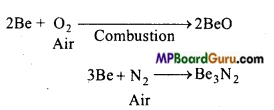Similarly magnesium is highly electropositive and bums in air with a bright light to form MgO and Mg3N2. Ca, Sr and Ba react readily with air to form oxide and nitride.
(ii) Reaction with hydrogen : Except Be, all other metals react with hydrogen on heating to form MH2 type of hydrides. (M = Mg, Ca, Sr, Ba)
(iii) Reaction with halogen : These metals react with halogens at high temperature to form MX2 type of halides.
M + X2 → MX2 (X = F, Cl, Br)

(iv) Reaction with acids : These metal react readily with acids and liberate H2 gas.
M+2HCl → MCl2 + H2
(v) Reducing nature : Like Alkali metals, alkaline earth metals are also of strong reducing nature, but less than alkali metals. On moving down the group their reducing nature decreases.
(vi) Like alkali metals, alkaline earth metals also dissolve in liquid ammonia to form bluish black solution.Question 20.
Compare the alkali metals and alkaline earth metals with respect to :
(i) Ionization enthalpy,
(ii) Basicity of oxides and
(iii) Solubility of hydroxides.
Answer:
(i) Ionization enthalpy : First ionization enthalpy of Alkaline earth metals is higher than that of corresponding alkali metals. But value of second ionization enthalpy of alkaline earth metals is less than the corresponding alkali metals.

(ii) Basicity of oxides: Oxides of alkali and alkaline earth metals dissolve in water to form basic hydroxides. Basicity of hydroxides of alkaline earth metals is less than that of hydroxides of alkali metals.
(iii) Solubility of hydroxides : Solubility of hydroxides of alkaline earth metals is less than that of corresponding alkali metals.

Question 21.
In what ways Lithium shows similarities to Magnesium in its chemical behaviour ?
Answer:
Due to similar size, Lithium and Magnesium show similar properties (Atomic radius Li = 152 pm, Mg = 160 pm).
Ionic radius : Li+ = 76 pm, Mg2+ = 72 pm. Their similar properties are as follows :
(i) Both Li and Mg are lighter and harder than other members of their group.
(ii) Both Li and Mg react slowly with water.
The s-Block Elements  Class 11 Important Questions Objective Type

1. Choose the correct answer:

Question 1.
Plaster of Paris is :
(a) (CaSO4)2.2H2O
(b) CaSO4.2H2O
(c) CaSO4.H2O
(d) CaSO4.
Answer:
(a) (CaSO4)2.2H2O

Question 2.
Lowest melting point compound :
(a) LiCl
(b) NaCl
(c) KCl
(d) RbCl.
Answer:
(a) LiCl

Question 3.
Active constituent of bleaching powder :
(a) CaOCl2
(b) Ca(OCl)Cl
(c) Ca(O2Cl2)
(d) CaCl2O2.
Answer:
(b) Ca(OCl)Cl

Question 4.
Solution of sodium in liquid ammonia is reducing due to following reason:
(a) Na atom
(b) NaH
(c) NaNH2
(d) e(NH3)x.
Answer:
(d) e(NH3)x.

Question 5.
Reaction of sodium with water is vigorous, not with lithium because lithium :
(a) Atomic mass is more
(b) Is a metal
(c) Is more electropositive
(d) Is more electronegative.
Answer:
(d) Is more electronegative.

Question 6.
Order of stability of various chlorides :
(a) LiCl > KC1 > NaCl > CsCl
(b) CsCl > KC1 > NaCl > LiCl
(c) NaCl > KC1 > LiCl > CsCl
(d) KCl>CsCl > NaCl > LiCl.
Answer:
(a) LiCl > KC1 > NaCl > CsClQuestion 7.
Hydration energy of Mg2+ ion will be more than :
(a) Al3+
(b) Na+
(c) Be2+
(d) Mg3+.
Answer:
(b) Na+

Question 8.
Which property of alkaline earth metals increase with increase in atomic number :
(a) Ionization energy
(b) Solubility of hydroxides
(c) Solubility of sulphates
(d) Electronegativity.
Answer:
(b) Solubility of hydroxides

Question 9.
Which is used for drying air :
(a) CaCO3
(b) Na2CO3
(c) NaHCO3
(d) CaO.
Answer:
(d) CaO.

Question 10.
Metallic lustre of sodium is due to :
(a) Diffusion of Na+ ion
(b) Rotation of electrons of outermost shell
(c) Excitation of free electrons Which sulphate has least solubility
(d) bec lattice.
Answer:
(c) Excitation of free electrons Which sulphate has least solubility

11. Which sulphate has least solubility:
(a) BaSO4
(b) MgSO4
(c) SrSO4
(d) CaSO4.
Answer:
(a) BaSO4

Question 12.
Order of thermal stability of carbonates of alkaline earth metals is :
(a) BaCO3 > SrCO3 > CaCO3 > MgCO3
(b) BaCO3 > SrCO3 > MgCO3 > CaCO3
(c) CaCO3 > SrCO3 > MgCO3 > BaCO3
(d) MgCO3 > CaCO3 > SrCO3 > BaCO3.
Answer:
(d) MgCO3 > CaCO3 > SrCO3 > BaCO3.

Question 13.
Compounds of which element are mainly covalent:
(a) Ba
(b) Sr
(c) Ca
(d) Be.
Answer:
(d) Be.

Question 14.
Important ore of magnesium is :
(a) Malachite
(b) Cassiterite
(c) Camallite
(d) Galena.
Answer:
(c) CamalliteQuestion 15.
Functions as air purifier in air craft:
(a) CaO
(b) Ca(OH)2
(c) KO2
(d) Anhydrous CaCl2.
Answer:
(c) KO2

Question 16.
Solubility of sulphates of alkaline earth metals decreases in moving down the group. Its reason is :
(a) Increase in melting point
(b) Increase in lattice energy
(c) Increase in coordination number
(d) All of these.
Answer:
(b) Increase in lattice energy

Question 17.
Which element does not give flame test:
(a) Be
(b) Ca
(c) Ba
(d) Sr.
Answer:
(a) Be

Question 18.
Which ore is not of lithium : ’
(a) Petalite
(b) Triphylite
(c) Elbite
(d) Spodumine.
Answer:
(c) Elbite

Question 19.
Magnesium is present in :
(a) Haemoglobin
(b) Chlorophyll
(c) Vitamin B12
(d) Vitamin C.
Answer:
(b) Chlorophyll

Question 20.
Mixture of MgCl2 and MgO is known as :
(a) Sorrel cement
(b) Portland cement
(c) Fitkari
(d) Mortar.
Answer:
(c) Fitkari

Question 21.
Mixture of CaCN2 and C is known as :
(a) Barytte
(b) Anhydrite
(c) Nitrolim
(d) Iceland spot.
Answer:
(a) Barytte

Question 22.
Lithopone is :
(a) BaSO4 + BaS
(b) BaSO4 + ZnS
(c) BaO + ZnS
(d) BaCO3 + ZnO.
Answer:
(b) BaSO4 + ZnS

Question 23.
Micro-cosmic salt is:
(a) Na(NH4) HPO4.4H2O
(b) Na(NH4)H2O.
(c) Na(NH3) HPO4.4H2O
(d) K(NH4)HPO3.2H2O.
Answer:
(a) Na(NH4) HPO4.4H2O

Question 24.
Which of the following alkali metals gives hydrated salts :
(a) Li
(b) Na
(c) K
(d) Cs.
Answer:
(a) Li

Question 25.
Which of the following alkali metals has lowest melting point:
(a) Na
(b) K
(c) Rb
(d) Cs.
Answer:
(d) Cs.

Question 26.
Which of the following alkaline earth metal carbonate is more stable towards heat:
(a) MgCO3
(b) CaCO3
(c) SrCO3
(d) BaCO3.
Answer:
(d) BaCO3.Question 27.
Co-product of Solvay ammonia process is :
(a) CO2
(b) NH3
(c) CaCl2
(d) CaCO3.
Answer:
(c) CaCl2

Question 28.
Increasing order of ionic product is :
(a) BeCl2 < MgCl2 < CaCl2 < BaCl2
(b) BeCl2 < MgCl2 < BaCl2 < CaCl2
(c) BeCl2 < BaCl2 < MgCl2 < CaCl2
(d) BaCl2 < CaCl2 < MgCl2 < BeCl2.
Answer:
(a) BeCl2 < MgCl2 < CaCl2 < BaCl2

Question 29.
Which of the following is paramagnetic :
(a) KO2
(b) SiO2
(c) TiO2
(d) BaO2.
Answer:
(a) KO2

2. Fill in the blanks:

1. Radius of Ca2+ ion is less than K+ because of ……………….. .
Answer:
High positive charge

2. Be(OH)2 is soluble in both acid and base because it is of ……………….. nature.
Answer:
Amphoteric

3. Lithium resemble ……………….. element of group 2.
Answer:
Mg

4. Sodium metal gives blue colour in liquid ammonia this is because of ……………….. .
Answer:
e (NH3)x

5. Potassium forms three oxides ……………….., ……………….. and ……………….. .
Answer:
K2O, K2O, KO2

6. Formula of hydrolith is ……………….. .
Answer:
CaH2

7. s-block elements are strong ……………….. .
Answer:
Reducing agent

8. Alkaline earth metal ……………….. represent radio-active property.
Answer:
Radium

9. Alkali metal ……………….. represent radio-active property.
Answer:
Francium (Fr)

10. Alkaline earth metal hydroxide ……………….. is amphoteric.
Answer:
Be(OH)2

11. Chemical formula of camallite is ……………….. .
Answer:
KCl.MgCl2.6H2O.

3. Match the following:

 ‘A’ ‘B’ 1. To dry air (a) Ca(OCI)C1 2. Nitrolim is (b) MgCl2 and MgO 3. Mixture of Sorrel cement (c) BaSO4 and ZnS 4. Active component of bleaching powder (d) CaCN2 and C 5. Mixture of Lithopone (e) CaO 6. CaCO3 (f) Smoke screen 7. SiCl4 (g) Limestone.

Answer:
1.  (e) CaO
2. (d) CaCN2 and C
3. (b) MgCl2 and MgO
4. (a) Ca(OCl)Cl
5. (c) BaSO4 and ZnS
6.  (g) Limestone
7. (f) Smoke screen.4. Answer in one word/sentence:

1. Arrange the alkaline earth metals in the order of their reactivity.
Answer:
Chemical reactivity of alkaline earth metals increases on moving down the group (Be-Ra).
Be < Mg < Ca < Sr < Ba < Ra.

2. Write name and formula of two ores of lithium.
Answer:
(i) Spodumene LiAl(SiO3)2,
(ii) Lepidolite Li2Al2(SiO3)3.F(OH)2.

3. Write names of two ores of magnesium.
Answer:
Two ores of magnesium are :
(i) Camallite
(ii) Dolomite.

4. Alkali metals are kept in kerosene oil. Why?
Answer:
Due to high reactivity.

5. Write the order of basic strength and solubility in water of hydroxides of alkaline earth metals.
Answer:
Basic strength and solubility of hydroxides of alkaline earth metals increases from top to bottom in a group.

6. Molecular formula of Glauber’s salt is.
Answer:
Na2SO4.10 H2O.

5. State true or false :

1. Alkali metals are not found in free state but alkaline earth metals exist in free state in earth.
Answer:
False

2. Basicity of hydroxides of alkali metals increases on moving down in the group.
Answer:
True

3. Soda ash means Baking soda.
Answer:
False

4. Magnesium does not impart any colour to Bunsen flame whereas calcium give flame test.
Answer:
True

5. Lil is less ionic as compared to Nal.
Answer:
False

6. Common salt wets because it has less hydration energy.
Answer:
False

7. Due to diagonal relationship properties of sodium are similar to calcium and that of caesium are similar to strontium.
Answer:
False

8. Francium is a radio-active element.
Answer:
True

9. For similar chemical properties sufficient difference in electronegativity is essential.
Answer:
False

10. Na2O is formed by heating sodium in excess of O2.
Answer:
False

11. Concentration of K+ and Na+ is same in blood.
Answer:
False
12.Concentration of Na+ is very less as compared to K+ concentration inside a cell.
True.
Answer: